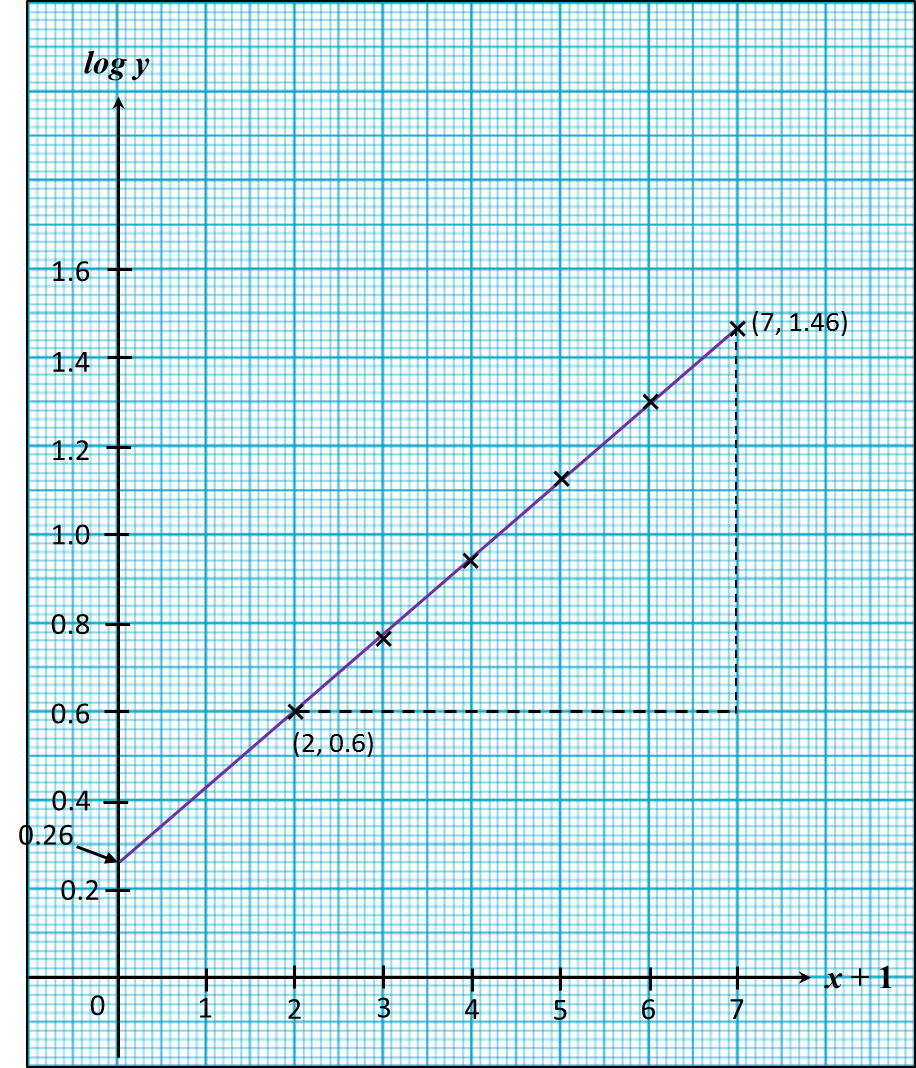# SPM Additional Mathematics (Model Test Paper) – Q&A 9-10

SPM Additional Mathematics (Model Test Paper)

Section B
[40 marks]
Answer any four questions from this section.

Question 9
Use graph paper to answer this question.
Table 1 shows the values of two variables, x and y, obtained from an experiment. The variables x and y are related by the equation y = hkx + 1, where h and k are constants.

 x 1 2 3 4 5 6 y 4 5.7 8.7 13.2 20 28.8
Table 1

(a) Based on table 1, construct a table for the values (x + 1) and log y. [2 marks]
(b) Plot log y against (x + 1), using a scale of 2 cm to 1 uint on the (x + 1) –axis and 2 cm to 0.2 unit on the log y-axis.
Hence, draw the line of best fit.    [3 marks]
(c) Use your graph in 9(b) to find the value of
(i) h.
(ii) k.  [5 marks]

(a)
 x + 1 2 3 4 5 6 7 log y 0.6 0.76 0.94 1.12 1.3 1.46

(b)(c)
= hkx + 1
log y = log h + (x + 1) log k
log y = log k (x + 1) + log h
Y = log y
m = log k
X = (x + 1)
c = log h

(i)
log h = y-intercept
log h = 0.26
h = 1.82

(ii)
$\begin{array}{l}\mathrm{log}k=\text{Gradient of the graph}\\ \text{}=\frac{1.46-0.60}{7-2}\\ \text{}=0.172\\ k=1.486\end{array}$

Question 10
(a) 20% of the students in SMK Bukit Bintang are cycling to school. If 9 pupils from the school are chosen at random, calculate the probability that
(i) exactly 3 of them are cycling to school,
(ii) at least a student is cycling to school. [4 marks]
(b) The volume of 800 bottles of fresh milk produced by a factory follows a normal distribution with a mean of 520 ml per bottle and variance of 1600 ml2.
(i) Find the probability that a bottle of fresh milk chosen in random has a volume of less than 515 ml.
(ii) If 480 bottles out of 800 bottles of the fresh milk have volume greater that k ml, find the value of k[6 marks]

(a)(i)
X~ Students in SMK Bukit Bintang who are cycling to school
X~ B (n, p)
X~ B (9, 0.2)
P (X = r) = nCr. pr. qn-r
Probability, exactly 3 students are cycling to school
(X = 3) = 9C3(0.2)3 (0.8)6
= 0.1761

(a)(ii)
At least a student is cycling to school
= 1 – (X = 0)
= 1 – 9C0 (0.2)0(0.8)9
= 0.8658

(b)(i)
m = 520 ml
σ= 1600 ml2
σ = 40
Let X represents volume of a bottle of fresh milk.
X ~ N (520, 1600)

(X < 515)
$=P\left(Z<\frac{515-520}{40}\right)$
= (Z< – 0.125)
= (Z> 0.125)
= 0.4502

(b)(ii)
$\begin{array}{l}P\left(X>k\right)=\frac{480}{800}\\ P\left(Z>\frac{k-520}{40}\right)=0.6\\ \frac{k-520}{40}=-0.253\\ k-520=-10.12\\ k=509.88\end{array}$

### 1 thought on “SPM Additional Mathematics (Model Test Paper) – Q&A 9-10”

1.perkongsian bijak, m
berguna untuk saya